## 1 Introduction

Monitoring consists in checking whether a sequence of data (a log or a signal) satisfies or violates a specification expressed using some formalism. Offline monitoring consists in performing this analysis after the system execution, as the technique has access to the entire log in order to decide whether the specification is violated. In contrast, online monitoring can make a decision earlier, ideally as soon as a witness of the violation of the specification is encountered.

Using existing formalisms (e.g., the metric first order temporal logic ), one can check whether a given bank customer withdraws more than 1,000€ every week. With formalisms extended with data, one may even identify such customers. Or, using an extension of the signal temporal logic (STL) , one can ask: “is that true that the value of variable x is always copied to y exactly 4 time units later?” However, questions relating time and data using parameters become much harder (or even impossible) to express using existing formalisms: “what are the users and time frames during which a user withdraws more than half of the total bank withdrawals within seven days?” And even, can we synthesize the durations (not necessarily 7 days) for which this specification holds? Or “what is the set of variables for which there exists a duration within which their value is always copied to another variable?” In addition, detecting periodic behaviors without knowing the period can be hard to achieve using existing formalisms.

In this work, we address the challenging problem to monitor logs enriched with both timing information and (infinite domain) data. In addition, we significantly push the existing limits of expressiveness so as to allow for a further level of abstraction using parameters: our specification can be both parametric in the time and in the data. The answer to this symbolic monitoring is richer than a pure Boolean answer, as it synthesizes the values of both time and data parameters for which the specification holds. This allows us notably to detect periodic behaviors without knowing the period while being symbolic in terms of data. For example, we can synthesize variable names (data) and delays for which variables will have their value copied to another data within the aforementioned delay. In addition, we show that we can detect the log segments (start and end date) for which a specification holds.

### Example 1

Consider a system updating three variables a, b and c (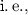strings) to values (rationals). An example of log is given in Fig. 1a. Although our work is event-based, we can give a graphical representation similar to that of signals in Fig. 1b. Consider the following property: “for any variable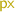, whenever an update of that variable occurs, then within strictly less than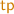time units, the value of variable b must be equal to that update”. The variable parameteris compared with string values and the timing parameteris used in the timing constraints. We are interested in checking for which values ofandthis property is violated. This can be seen as a synthesis problem in both the variable and timing parameters. For example,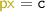and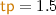is a violation of the specification, as the update of $$\mathtt {c}$$ to 2 at time 4 is not propagated to $$\mathtt {b}$$ within 1.5 time unit. Our algorithm outputs such violation by a constraint e.g.,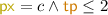. In contrast, the value of any signal at any time is always such that either b is equal to that signal, or the value of b will be equal to that value within at most 2 time units. Thus, the specification holds for any valuation of the variable parameter, provided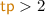.

We propose an automata-based approach to perform monitoring parametric in both time and data. We implement our work in a prototype SyMon and perform experiments showing that, while our formalism allows for high expressiveness, it is also tractable even for online monitoring.

We believe our framework balances expressiveness and monitoring performance well: (i) Regarding expressiveness, comparison with the existing work is summarized in Table 1 (see Sect. 2 for further details). (ii) Our monitoring is complete, in the sense that it returns a symbolic constraint characterizing all the parameter valuations that match a given specification. (iii) We also achieve reasonable monitoring speed, especially given the degree of parametrization in our formalism. Note that it is not easy to formally claim superiority in expressiveness: proofs would require arguments such as the pumping lemma; and such formal comparison does not seem to be a concern of the existing work. Moreover, such formal comparison bears little importance for industrial practitioners: expressivity via an elaborate encoding is hardly of practical use. We also note that, in the existing work, we often observe gaps between the formalism in a theory and the formalism that the resulting tool actually accepts. This is not the case with the current framework.

Outline. After discussing related works in Sect. 2, we introduce the necessary preliminaries in Sect. 3, and our parametric timed data automata in Sect. 4. We present our symbolic monitoring approach in Sect. 5 and conduct experiments in Sect. 6. We conclude in Sect. 7.

## 2 Related Works

Robustness and Monitoring. Robust (or quantitative) monitoring extends the binary question whether a log satisfies a specification by asking “by how much” the specification is satisfied. The quantification of the distance between a signal and a signal temporal logic (STL) specification has been addressed in, e.g., [20,21,22,23, 25, 27] (or in a slightly different setting in ). The distance can be understood in terms of space (“signals”) or time. In , the distance also copes for reordering of events. In , the robust pattern matching problem is considered over signal regular expressions, by quantifying the distance between the signal regular expression specification and the segments of the signal. For piecewise-constant and piecewise-linear signals, the problem can be effectively solved using a finite union of convex polyhedra. While our framework does not fit in robust monitoring, we can simulate both the robustness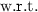time (using timing parameters) and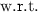data, e.g., signal values (using data parameters).

Monitoring with Data. The tool MarQ  performs monitoring using Quantified Event Automata (QEA) . This approach and ours share the automata-based framework, the ability to express some first-order properties using “events containing data” (which we encode using local variables associated with actions), and data may be quantified. However,  does not seem to natively support specification parametric in time; in addition,  does not perform complete (“symbolic”) parameters synthesis, but outputs the violating entries of the log.

The metric first order temporal logic (MFOTL) allows for a high expressiveness by allowing universal and existential quantification over data—which can be seen as a way to express parameters. A monitoring algorithm is presented for a safety fragment of MFOTL in . Aggregation operators are added in , allowing to compute sums or maximums over data. A fragment of this logics is implemented in MonPoly . While these works are highly expressive, they do not natively consider timing parameters; in addition, MonPoly does not output symbolic answers,symbolic conditions on the parameters to ensure validity of the formula.

In , binary decision diagrams (BDDs) are used to symbolically represent the observed data in QTL. This can be seen as monitoring data against a parametric specification, with a symbolic internal encoding. However, their implementation DejaVu only outputs concrete answers. In contrast, we are able to provide symbolic answers (both in timing and data parameters), e.g., in the form of union of polyhedra for rationals, and unions of string constraints using equalities ($$=$$) and inequalities ($$\ne$$).

Freeze Operator. In , STL is extended with a freeze operator that can “remember” the value of a signal, to compare it to a later value of the same signal. This logic STL$$^*$$ can express properties such as “In the initial 10 s, x copies the values of y within a delay of 4 s”: $$\mathbf {G}_{[0,10]} *( \mathbf {G}_{[0, 4]} y^*= x )$$. While the setting is somehow different (STL$$^*$$ operates over signals while we operate over timed data words), the requirements such as the one above can easily be encoded in our framework. In addition, we are able to synthesize the delay within which the values are always copied, as in Example 1. In contrast, it is not possible to determine using STL$$^*$$ which variables and which delays violate the specification.

Monitoring with Parameters. In , a log in the form of a dense-time real-valued signal is tested against a parameterized extension of STL, where parameters can be used to model uncertainty both in signal values and in timing values. The output comes in the form of a subset of the parameters space for which the formula holds on the log. In , the focus is only on signal parameters, with an improved efficiency by reusing techniques from the robust monitoring. Whereas [7, 9] fit in the framework of signals and temporal logics while we fit in words and automata, our work shares similarities with [7, 9] in the sense that we can express data parameters; in addition,  is able as in our work to exhibit the segment of the log associated with the parameters valuations for which the specification holds. A main difference however is that we can use memory and aggregation, thanks to arithmetic on variables.

In , the problem of inferring temporal logic formulae with constraints that hold in a given numerical data time series is addressed.

Timed Pattern Matching. A recent line of work is that of timed pattern matching, that takes as input a log and a specification, and decides where in the log the specification is satisfied or violated. On the one hand, a line of works considers signals, with specifications either in the form of timed regular expressions [11, 31,32,33], or a temporal logic . On the other hand, a line of works considers timed words, with specifications in the form of timed automata [4, 36]. We will see that our work can also encode parametric timed pattern matching. Therefore, our work can be seen as a two-dimensional extension of both lines of works: first, we add timing parameters ( also considers similar timing parameters) and, second, we add data—themselves extended with parameters. That is, coming back to Example 1, [31,32,33, 36] could only infer the segments of the log for which the property is violated for a given (fixed) variable and a given (fixed) timing parameter; while  could infer both the segments of the log and the timing parameter valuations, but not which variable violates the specification.

Summary. We compare related works in Table 1. “Timing parameters” denote the ability to synthesize unknown constants used in timing constraints (e.g., modalities intervals, or clock constraints). “” denotes works not natively supporting this, although it might be encoded. The term “Data” refers to the ability to manage logs over infinite domains (apart from timestamps). For example, the log in Fig. 1a features, beyond timestamps, both string (variable name) and rationals (value). Also, works based on real-valued signals are naturally able to manage (at least one type of) data. “Parametric data” refer to the ability to express formulas where data (including signal values) are compared to (quantified or unquantified) variables or unknown parameters; for example, in the log in Fig. 1a, an example of property parametric in data is to synthesize the parameters for which the difference of values between two consecutive updates of variableis always below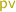, whereis a string parameter anda rational-valued parameter. “Memory” is the ability to remember past data; this can be achieved using e.g., the freeze operator of STL$$^*$$, or variables (e.g., in [14, 26, 30]). “Aggregation” is the ability to aggregate data using operators such as sum or maximum; this allows to express properties such as “A user must not withdraw more than \$10,000 within a 31 day period” . This can be supported using dedicated aggregation operators  or using variables (, and our work). “Complete parameter identification” denotes the synthesis of the set of parameters that satisfy or violate the property. Here, “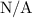” denotes the absence of parameter , or when parameters are used in a way (existentially or universally quantified) such as the identification is not explicit (instead, the position of the log where the property is violated is returned ). In contrast, we return in a symbolic manner (as in [4, 7]) the exact set of (data and timing) parameters for which a property is satisfied. “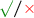” denotes “yes” in the theory paper, but not in the tool.

## 3 Preliminaries

Clocks, Timing Parameters and Timed Guards. We assume a set $$\mathbb {C}= \{ c_1, \dots , c_H\}$$ of clocks,real-valued variables that evolve at the same rate. A clock valuation is $$\nu : \mathbb {C}\rightarrow {\mathbb {R}}_{\ge 0}$$. We write $$\mathbf {0}$$ for the clock valuation assigning 0 to all clocks. Given $$d \in {\mathbb {R}}_{\ge 0}$$, $$\nu + d$$ is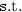$$(\nu + d)(c) = \nu (c) + d$$, for all $$c\in \mathbb {C}$$. Given $$R\subseteq \mathbb {C}$$, we define the reset of a valuation $$\nu$$, denoted by $$[\nu ]_{R}$$, as follows: $$[\nu ]_{R}(c) = 0$$ if $$c\in R$$, and $$[\nu ]_{R}(c)=\nu (c)$$ otherwise.

We assume a set $$\mathbb {TP}= \{ \mathsf {tp}_1, \dots , \mathsf {tp}_J\}$$ of timing parameters. A timing parameter valuation is $$\gamma : \mathbb {TP}\rightarrow {\mathbb Q}_{+}$$. We assume $${\bowtie } \in \{<, \le , =, \ge , >\}$$. A timed guard $$tg$$ is a constraint over $$\mathbb {C}\cup \mathbb {TP}$$ defined by a conjunction of inequalities of the form $$c\bowtie d$$, or $$c\bowtie \mathsf {tp}$$ with $$d \in {\mathbb N}$$ and $$\mathsf {tp}\in \mathbb {TP}$$. Given $$tg$$, we write $$\nu \models \gamma (tg)$$ if the expression obtained by replacing each $$c$$ with $$\nu (c)$$ and each $$\mathsf {tp}$$ with $$\gamma (\mathsf {tp})$$ in $$tg$$ evaluates to true.

Variables, Data Parameters and Data Guards. For sake of simplicity, we assume a single infinite domain $$\mathbb {D}$$ for data. The formalism defined in Sect. 4 can be extended in a straightforward manner to different domains for different variables (and our implementation does allow for different types). The case of finite data domain is immediate too. We define this formalism in an abstract manner, so as to allow a sort of parameterized domain.

We assume a set $$\mathbb {V}= \{ v_1, \dots , v_M\}$$ of variables valued over $$\mathbb {D}$$. These variables are internal variables, that allow an high expressive power in our framework, as they can be compared or updated to other variables or parameters. We also assume a set $$\mathbb {LV}= \{ lv _1, \dots , lv _O\}$$ of local variables valued over $$\mathbb {D}$$. These variables will only be used locally along a transition in the “argument” of the action (e.g.,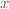andin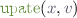), and in the associated guard and (right-hand part of) updates. We assume a set $$\mathbb {VP}= \{ \mathsf {vp}_1, \dots , \mathsf {vp}_N\}$$ of data parameters,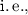unknown variable constants.

A data type $$(\mathbb {D}, \mathcal {DE}, \mathcal {DU})$$ is made of (i) an infinite domain $$\mathbb {D}$$, (ii) a set of admissible Boolean expressions $$\mathcal {DE}$$ (that may rely on $$\mathbb {V}$$, $$\mathbb {LV}$$ and $$\mathbb {VP}$$), which will define the type of guards over variables in our subsequent automata, and (iii) a domain for updates $$\mathcal {DU}$$ (that may rely on $$\mathbb {V}$$, $$\mathbb {LV}$$ and $$\mathbb {VP}$$), which will define the type of updates of variables in our subsequent automata.

### Example 2

As a first example, let us define the data type for rationals. We have $$\mathbb {D}= {\mathbb Q}$$. Let us define Boolean expressions. A rational comparison is a constraint over $$\mathbb {V}\cup \mathbb {LV}\cup \mathbb {VP}$$ defined by a conjunction of inequalities of the form $$v\bowtie d$$, $$v\bowtie v'$$, or $$v\bowtie \mathsf {vp}$$ with $$v, v' \in \mathbb {V}\cup \mathbb {LV}$$, $$d \in {\mathbb Q}$$ and $$\mathsf {vp}\in \mathbb {VP}$$. $$\mathcal {DE}$$ is the set of all rational comparisons over $$\mathbb {V}\cup \mathbb {LV}\cup \mathbb {VP}$$. Let us then define updates. First, a linear arithmetic expression over $$\mathbb {V}\cup \mathbb {LV}\cup \mathbb {VP}$$ is $$\sum _i \alpha _i v_i + \beta$$, where $$v_i \in \mathbb {V}\cup \mathbb {LV}\cup \mathbb {VP}$$ and $$\alpha _i, \beta \in {\mathbb Q}$$. Let $$\mathcal {LA}(\mathbb {V}\cup \mathbb {LV}\cup \mathbb {VP})$$ denote the set of arithmetic expressions over $$\mathbb {V}$$, $$\mathbb {LV}$$ and $$\mathbb {VP}$$. We then have $$\mathcal {DU}= \mathcal {LA}(\mathbb {V}\cup \mathbb {LV}\cup \mathbb {VP})$$.

As a second example, let us define the data type for strings. We have $$\mathbb {D}= {\mathbb S}$$, where $${\mathbb S}$$ denotes the set of all strings. A string comparison is a constraint over $$\mathbb {V}\cup \mathbb {LV}\cup \mathbb {VP}$$ defined by a conjunction of comparisons of the form $$v\approx s$$, $$v\approx v'$$, or $$v\approx \mathsf {vp}$$ with $$v, v' \in \mathbb {V}\cup \mathbb {LV}$$, $$s \in {\mathbb S}$$, $$\mathsf {vp}\in \mathbb {VP}$$ and $${\approx } \in \{ =, \ne \}$$. $$\mathcal {DE}$$ is the set of all string comparisons over $$\mathbb {V}\cup \mathbb {LV}\cup \mathbb {VP}$$. $$\mathcal {DU}= \mathbb {V}\cup \mathbb {LV}\cup {\mathbb S}$$,a string variable can be assigned another string variable, or a concrete string.

A variable valuation is $$\mu : \mathbb {V}\rightarrow \mathbb {D}$$. A local variable valuation is a partial function $$\eta : \mathbb {LV}\nrightarrow \mathbb {D}$$. A data parameter valuation is $$\zeta : \mathbb {VP}\rightarrow \mathbb {D}$$. Given a data guard $$dg\in \mathcal {DE}$$, a variable valuation $$\mu$$, a local variable valuation $$\eta$$ defined for the local variables in $$dg$$, and a data parameter valuation $$\zeta$$, we write $$(\mu , \eta ) \models \zeta (dg)$$ if the expression obtained by replacing within $$dg$$ all occurrences of each data parameter $$\mathsf {vp}_i$$ by $$\zeta (\mathsf {vp}_i)$$ and all occurrences of each variable $$v_j$$ (resp. local variable $$lv _k$$) with its concrete valuation $$\mu (v_j)$$ (resp. $$\eta ( lv _k)$$)) evaluates to true.

A parametric data update is a partial function $$\mathsf {PDU}: \mathbb {V}\nrightarrow \mathcal {DU}$$. That is, we can assign to a variable an expression over data parameters and other variables, according to the data type. Given a parametric data update $$\mathsf {PDU}$$, a variable valuation $$\mu$$, a local variable valuation $$\eta$$ (defined for all local variables appearing in $$\mathsf {PDU}$$), and a data parameter valuation $$\zeta$$, we define $$[\mu ]_{\eta (\zeta (\mathsf {PDU}))} : \mathbb {V}\rightarrow \mathbb {D}$$ as:

\begin{aligned}{}[\mu ]_{\eta (\zeta (\mathsf {PDU}))}(v) = {\left\{ \begin{array}{ll} \mu (v) &{} \text {if }\mathsf {PDU}(v) \text { is undefined} \\ \eta (\mu (\zeta (\mathsf {PDU}(v)))) &{} \text {otherwise} \\ \end{array}\right. } \end{aligned}

where $$\eta (\mu (\zeta (\mathsf {PDU}(v))))$$ denotes the replacement within the update expression $$\mathsf {PDU}(v)$$ of all occurrences of each data parameter $$\mathsf {vp}_i$$ by $$\zeta (\mathsf {vp}_i)$$, and all occurrences of each variable $$v_j$$ (resp. local variable $$lv _k$$) with its concrete valuation $$\mu (v_j)$$ (resp. $$\eta ( lv _k)$$). Observe that this replacement gives a value in $$\mathbb {D}$$, therefore the result of $$[\mu ]_{\eta (\zeta (\mathsf {PDU}))}$$ is indeed a data parameter valuation $$\mathbb {V}\rightarrow \mathbb {D}$$. That is, $$[\mu ]_{\eta (\zeta (\mathsf {PDU}))}$$ computes the new (non-parametric) variable valuation obtained after applying to $$\mu$$ the partial function $$\mathsf {PDU}$$ valuated with $$\zeta$$.

### Example 3

Consider the data type for rationals, the variables set $$\{ v_1, v_2 \}$$, the local variables set $$\{ lv _1, lv _2 \}$$ and the parameters set $$\{ \mathsf {vp}_1 \}$$. Let $$\mu$$ be the variable valuation such that $$\mu (v_1) = 1$$ and $$\mu (v_2) = 2$$, and $$\eta$$ be the local variable valuation such that $$\eta ( lv _1) = 2$$ and $$\eta ( lv _2)$$ is not defined. Let $$\zeta$$ be the data parameter valuation such that $$\zeta (\mathsf {vp}_1) = 1$$. Consider the parametric data update function $$\mathsf {PDU}$$ such that $$\mathsf {PDU}(v_1) = 2 \times v_1 + v_2 - lv _1 + \mathsf {vp}_1$$, and $$\mathsf {PDU}(v_2)$$ is undefined. Then the result of $$[\mu ]_{\eta (\zeta (\mathsf {PDU}))}$$ is $$\mu '$$ such that $$\mu '(v_1) = 2 \times \mu (v_1) + \mu (v_2) - \eta ( lv _1) + \zeta (\mathsf {vp}_1) = 3$$ and $$\mu '(v_2) = 2$$.

## 4 Parametric Timed Data Automata

We introduce here Parametric timed data automata (PTDAs). They can be seen as an extension of parametric timed automata  (that extend timed automata  with parameters in place of integer constants) with unbounded data variables and parametric variables. PTDAs can also be seen as an extension of some extensions of timed automata with data (see e.g., [16, 19, 29]), that we again extend with both data parameters and timing parameters. Or as an extension of quantified event automata  with explicit time representation using clocks, and further augmented with timing parameters. PTDAs feature both timed guards and data guards; we summarize the various variables and parameters types together with their notations in Table 2.

We will associate local variables with actions (which can be see as predicates). Let $$Dom : \varSigma \rightarrow 2^\mathbb {LV}$$ denote the set of local variables associated with each action. Let $$Var (dg)$$ (resp. $$Var (\mathsf {PDU})$$) denote the set of variables occurring in $$dg$$ (resp. $$\mathsf {PDU}$$).

### Definition 1

(PTDA). Given a data type $$(\mathbb {D}, \mathcal {DE}, \mathcal {DU})$$, a parametric timed data automaton (PTDA) $$\mathcal {A}$$ over this data type is a tuple $$\mathcal {A}= (\varSigma , L, \ell _0, F, \mathbb {C}, \mathbb {TP}, \mathbb {V}, \mathbb {LV}, \mu _0, \mathbb {VP}, E)$$, where:

1. 1.

$$\varSigma$$ is a finite set of actions,

2. 2.

$$L$$ is a finite set of locations, $$\ell _0\in L$$ is the initial location,

3. 3.

$$F\subseteq L$$ is the set of accepting locations,

4. 4.

$$\mathbb {C}$$ is a finite set of clocks,

5. 5.

$$\mathbb {TP}$$ is a finite set of timing parameters,

6. 6.

$$\mathbb {V}$$ (resp. $$\mathbb {LV}$$) is a finite set of variables (resp. local variables) over $$\mathbb {D}$$,

7. 7.

$$\mu _0$$ is the initial variable valuation,

8. 8.

$$\mathbb {VP}$$ is a finite set of data parameters,

9. 9.

$$E$$ is a finite set of edges $$e= (\ell , tg, dg, a, R, \mathsf {PDU}, \ell ')$$ where (i) $$\ell , \ell '\in L$$ are the source and target locations, (ii) $$tg$$ is a timed guard, (iii) $$dg\in \mathcal {DE}$$ is a data guard such as $$Var (dg) \cap \mathbb {LV}\subseteq Dom (a)$$, (iv) $$a\in \varSigma$$, (v) $$R\subseteq \mathbb {C}$$ is a set of clocks to be reset, and (vi) $$\mathsf {PDU}: \mathbb {V}\nrightarrow \mathcal {DU}$$ is the parametric data update function such that $$Var (\mathsf {PDU}) \cap \mathbb {LV}\subseteq Dom (a)$$.

The domain conditions on $$dg$$ and $$\mathsf {PDU}$$ ensure that the local variables used in the guard (resp. update) are only those in the action signature $$Dom (a)$$.

### Example 4

Consider the PTDA in Fig. 2b over the data type for strings. We have $$\mathbb {C}= \{ c\}$$, $$\mathbb {TP}= \{ \mathsf {tp}\}$$, $$\mathbb {V}= \emptyset$$ and $$\mathbb {LV}= \{ f , m \}$$.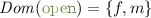while. $$\ell _2$$ is the only accepting location, modeling the violation of the specification.

This PTDA (freely inspired by a formula from  further extended with timing parameters) monitors the improper file opening and closing,a file already open should not be open again, and a file that is open should not be closed too late. The data parameter $$\mathsf {vp}$$ is used to symbolically monitor a given file name,we are interested in opening and closings of this file only, while other files are disregarded (specified using the self-loops in $$\ell _0$$ and $$\ell _1$$ with data guard $$f \ne \mathsf {vp}$$). Whenever f is opened (transition from $$\ell _0$$ to $$\ell _1$$), a clock $$c$$ is reset. Then, in $$\ell _1$$, if f is closed within $$\mathsf {tp}$$ time units (timed guard “$$c\le \mathsf {tp}$$”), then the system goes back to $$\ell _0$$. However, if instead f is opened again, this is an incorrect behavior and the system enters $$\ell _2$$ via the upper transition. The same occurs if f is closed more than $$\mathsf {tp}$$ time units after opening.

Given a data parameter valuation $$\zeta$$ and a timing parameter valuation $$\gamma$$, we denote by $$\gamma |\zeta (\mathcal {A})$$ the resulting timed data automaton (TDA),the non-parametric structure where all occurrences of a parameter $$\mathsf {vp}_i$$ (resp. $$\mathsf {tp}_j$$) have been replaced by $$\zeta (\mathsf {vp}_i)$$ (resp. $$\gamma (\mathsf {tp}_j)$$). Note that, if $$\mathbb {V}= \mathbb {LV}= \emptyset$$, then $$\mathcal {A}$$ is a parametric timed automaton  and $$\gamma |\zeta (\mathcal {A})$$ is a timed automaton .

We now equip our TDAs with a concrete semantics.

### Definition 2

(Semantics of a TDA). Given a PTDA $$\mathcal {A}= (\varSigma , L, \ell _0, F, \mathbb {C}, \mathbb {TP}, \mathbb {V}, \mathbb {LV}, \mu _0, \mathbb {VP}, E)$$ over a data type $$(\mathbb {D}, \mathcal {DE}, \mathcal {DU})$$, a data parameter valuation $$\zeta$$ and a timing parameter valuation $$\gamma$$, the semantics of $$\gamma |\zeta (\mathcal {A})$$ is given by the timed transition system (TTS) $$(S, s_0, {\rightarrow })$$, with

• $$S= L\times \mathbb {D}^M\times {\mathbb {R}}_{\ge 0}^H$$, $$s_0= (\ell _0, \mu _0, \mathbf {0})$$,

• $${\rightarrow }$$ consists of the discrete and (continuous) delay transition relations:

1. 1.

discrete transitions: $$(\ell , \mu , \nu ) {\mathop {\mapsto }\limits ^{e, \eta }} (\ell ', \mu ', \nu ')$$, there exist $$e= (\ell , tg, dg, a, R, \mathsf {PDU}, \ell ') \in E$$ and a local variable valuation $$\eta$$ defined exactly for $$Dom (a)$$, such that $$\nu \models \gamma (tg$$), $$(\mu , \eta ) \models \zeta (dg)$$, $$\nu ' = [\nu ]_{R}$$, and $$\mu ' = [\mu ]_{\eta (\zeta (\mathsf {PDU}))}$$.

2. 2.

delay transitions: $$(\ell , \mu , \nu ) {\mathop {\mapsto }\limits ^{d}} (\ell , \mu , \nu + d)$$, with $$d \in {\mathbb {R}}_{\ge 0}$$.

Moreover we write $$((\ell , \mu , \nu ) , (e, \eta , d) , (\ell ', \mu ', \nu ')) \in {\rightarrow }$$ for a combination of a delay and discrete transition if $$\exists \nu '' : (\ell , \mu , \nu ) {\mathop {\mapsto }\limits ^{d}} (\ell , \mu , \nu '') {\mathop {\mapsto }\limits ^{e, \eta }} (\ell ', \mu ', \nu ')$$.

Given a TDA $$\gamma |\zeta (\mathcal {A})$$ with concrete semantics $$(S, s_0, {\rightarrow })$$, we refer to the states of $$S$$ as the concrete states of $$\gamma |\zeta (\mathcal {A})$$. A run of $$\gamma |\zeta (\mathcal {A})$$ is an alternating sequence of concrete states of $$\gamma |\zeta (\mathcal {A})$$ and triples of edges, local variable valuations and delays, starting from the initial state $$s_0$$ of the form $$(\ell _0, \mu _0, \nu _0), (e_0, \eta , d_0), (\ell _1, \mu _1, \nu _1), \cdots$$ with $$i = 0, 1, \dots$$, $$e_i \in E$$, $$d_i \in {\mathbb {R}}_{\ge 0}$$ and $$((\ell _i, \mu _i, \nu _i) , (e_i , \eta _i, d_i) , (\ell _{i+1}, \mu _{i+1}, \nu _{i+1})) \in {\rightarrow }$$. Given such a run, the associated timed data word is $$(a_1, \tau _1, \eta _1), (a_2, \tau _2, \eta _2), \cdots$$, where $$a_i$$ is the action of edge $$e_{i-1}$$, $$\eta _i$$ is the local variable valuation associated with that transition, and $$\tau _i = \sum _{0 \le j \le i-1} d_j$$, for $$i = 1, 2 \cdots$$. For a timed data word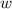and a concrete state $$(\ell ,\mu ,\nu )$$ of $$\gamma |\zeta (\mathcal {A})$$, we write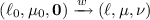in $$\gamma |\zeta (\mathcal {A})$$ ifis associated with a run of $$\gamma |\zeta (\mathcal {A})$$ of the form $$(\ell _0, \mu _0, \mathbf {0}), \dots , (\ell _n,\mu _n,\nu _n)$$ with $$(\ell _n,\mu _n,\nu _n) = (\ell ,\mu ,\nu )$$. For a timed data word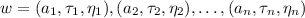, we denote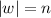and for any $$i \in \{1,2,\dots ,n\}$$, we denote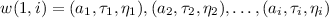.

A finite run is accepting if its last state $$(\ell , \mu , \nu )$$ is such that $$\ell \in F$$. The language $$\mathcal {L}(\gamma |\zeta (\mathcal {A}))$$ is defined to be the set of timed data words associated with all accepting runs of $$\gamma |\zeta (\mathcal {A})$$.

### Example 5

Consider the PTDA in Fig. 2b over the data type for strings. Let $$\gamma (\mathsf {tp}) = 100$$ and $$\zeta (\mathsf {vp}) = \mathtt {Hakuchi{.}txt}$$. An accepting run of the TDA $$\gamma |\zeta (\mathcal {A})$$ is: $$(\ell _0, \emptyset , \nu _0), (e_0, \eta _0, 2046), (\ell _1, \emptyset , \nu _1), (e_1, \eta _1, 90), (\ell _1, \emptyset , \nu _2) (e_2, \eta _2, 30), (\ell _2, \emptyset , \nu _3)$$, where $$\emptyset$$ denotes a variable valuation over an empty domain (recall that $$\mathbb {V}= \emptyset$$ in Fig. 2b), $$\nu _0(c) = 0$$, $$\nu _1(c) = 0$$, $$\nu _2(c) = 90$$, $$\nu _3(c) = 120$$, $$e_0$$ is the upper edge from $$\ell _0$$ to $$\ell _1$$, $$e_1$$ is the self-loop above $$\ell _1$$, $$e_2$$ is the lower edge from $$\ell _1$$ to $$\ell _2$$, $$\eta _0(f) = \eta _2(f) = \mathtt {Hakuchi{.}txt}$$, $$\eta _1(f) = \mathtt {Unagi.mp4}$$, $$\eta _0(m) = \eta _1(m) = \mathtt {rw}$$, and $$\eta _2(m)$$ is undefined (because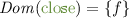).

The associated timed data word is.

Since each action is associated with a set of local variables, given an ordering on this set, it is possible to see a given action and a variable valuation as a predicate: for example, assuming an ordering of $$\mathbb {LV}$$ such as f precedes m, then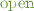with $$\eta _0$$ can be represented as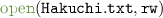. Using this convention, the log in Fig. 2a corresponds exactly to this timed data word.

## 5 Symbolic Monitoring Against PTDA Specifications

In symbolic monitoring, in addition to the (observable) actions in $$\varSigma$$, we employ unobservable actions denoted by $$\varepsilon$$ and satisfying $$Dom (\varepsilon ) = \emptyset$$. We write $$\varSigma _{\varepsilon }$$ for $$\varSigma \sqcup \{\varepsilon \}$$. We let $$\eta _{\varepsilon }$$ be the local variable valuation such that $$\eta _{\varepsilon }( lv )$$ is undefined for any $$lv \in \mathbb {LV}$$. For a timed data word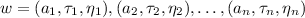over $$\varSigma _{\varepsilon }$$, the projection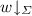is the timed data word over $$\varSigma$$ obtained fromby removing any triple $$(a_i,\tau _i,\eta _i)$$ where $$a_i = \varepsilon$$. An edge $$e= (\ell , tg, dg, a, R, \mathsf {PDU}, \ell ') \in E$$ is unobservable if $$a= \varepsilon$$, and observable otherwise. The use of unobservable actions allows us to encode parametric timed pattern matching (see Sect. 5.3).

We make the following assumption on the PTDAs in symbolic monitoring.

### Assumption 1

The PTDA $$\mathcal {A}$$ does not contain any loop of unobservable edges.

### 5.1 Problem Definition

Roughly speaking, given a PTDA $$\mathcal {A}$$ and a timed data word, the symbolic monitoring problem asks for the set of pairs $$(\gamma , \zeta ) \in ({{\mathbb Q}_{+}})^{\mathbb {TP}}\times \mathbb {D}^\mathbb {VP}$$ satisfying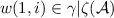, where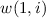is a prefix of. Since $$\mathcal {A}$$ also contains unobservable edges, we consider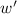which isaugmented by unobservable actions.

### Example 6

Consider the PTDA $$\mathcal {A}$$ and the timed data wordshown in Fig. 1. The validity domain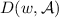is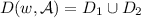, where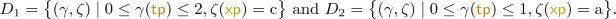For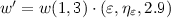, we have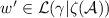and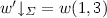, where $$\gamma$$ and $$\zeta$$ are such that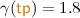and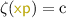, and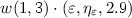denotes the juxtaposition.

For the data types in Example 2, the validity domain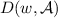can be represented by a constraint of finite size because the length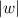of the timed data word is finite.

### 5.2 Online Algorithm

Our algorithm is online in the sense that it outputs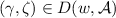as soon as its membership is witnessed, even before reading the whole timed data word.

Let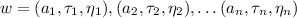and $$\mathcal {A}$$ be the timed data word and PTDA given in symbolic monitoring, respectively. Intuitively, after reading $$(a_i,\tau _i,\eta _i)$$, our algorithm symbolically computes for all parameter valuations $$(\gamma ,\zeta ) \in ({{\mathbb Q}_{+}})^{\mathbb {TP}}\times \mathbb {D}^{\mathbb {VP}}$$ the concrete states $$(\ell ,\nu ,\mu )$$ satisfying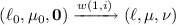in $$\gamma |\zeta (\mathcal {A})$$. Since $$\mathcal {A}$$ has unobservable edges as well as observable edges, we have to add unobservable actions before or after observable actions in. By $$Conf ^{o}_{i}$$, we denote the configurations after reading $$(a_i,\tau _i,\eta _i)$$ and no unobservable actions are appended after $$(a_i,\tau _i,\eta _i)$$. By $$Conf ^{u}_{i}$$, we denote the configurations after reading $$(a_i,\tau _i,\eta _i)$$ and at least one unobservable action is appended after $$(a_i,\tau _i,\eta _i)$$.

### Definition 3

$$\mathbf{(} Conf ^{o}_{i}, Conf ^{u}_{i}{} \mathbf{).}$$ For a PTDA $$\mathcal {A}$$ over actions $$\varSigma _{\varepsilon }$$, a timed data wordover $$\varSigma$$, and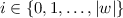(resp.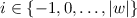), $$Conf ^{o}_{i}$$ (resp. $$Conf ^{u}_{i}$$) is the set of 5-tuples $$(\ell ,\nu ,\gamma ,\mu ,\zeta )$$ such that there is a timed data word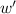over $$\varSigma _{\varepsilon }$$ satisfying the following: (i)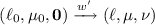in $$\gamma |\zeta (\mathcal {A})$$, (ii)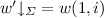, (iii) The last action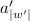ofis observable (resp. unobservable and its timestamp is less than $$\tau _{i+1}$$).

Algorithm 1 shows an outline of our algorithm for symbolic monitoring (see  for the full version). Our algorithm incrementally computes $$Conf ^{u}_{i-1}$$ and $$Conf ^{o}_{i}$$ (line 3). After reading $$(a_i,\tau _i,\eta _i)$$, our algorithm stores the partial resultswitnessed from the accepting configurations in $$Conf ^{u}_{i-1}$$ and $$Conf ^{o}_{i}$$ (line 4). (We also need to try to take potential unobservable transitions and store the results from the accepting configurations after the last element of the timed data word (lines 5 and 6).)

Since $$({{\mathbb Q}_{+}})^{\mathbb {TP}}\times \mathbb {D}^{\mathbb {VP}}$$ is an infinite set, we cannot try each $$(\gamma , \zeta ) \in ({{\mathbb Q}_{+}})^{\mathbb {TP}}\times \mathbb {D}^{\mathbb {VP}}$$ and we use a symbolic representation for parameter valuations. Similarly to the reachability synthesis of parametric timed automata , a set of clock and timing parameter valuations can be represented by a convex polyhedron. For variable valuations and data parameter valuations, we need an appropriate representation depending on the data type $$(\mathbb {D}, \mathcal {DE}, \mathcal {DU})$$. Moreover, for the termination of Algorithm 1, some operations on the symbolic representation are required.

### Theorem 1

(termination). For any PTDA $$\mathcal {A}$$ over a data type $$(\mathbb {D}, \mathcal {DE}, \mathcal {DU})$$ and actions $$\varSigma _{\varepsilon }$$, and for any timed data wordover $$\varSigma$$, Algorithm 1 terminates if the following operations on the symbolic representation $$V_{d}$$ of a set of variable and data parameter valuations terminate.

1. 1.

restriction and update $$\{ ([\mu ]_{\eta (\zeta (\mathsf {PDU}))}, \zeta ) \mid \exists (\mu ,\zeta )\in V_{d}.\, (\mu , \eta ) \models \zeta (dg) \}$$, where $$\eta$$ is a local variable valuation, $$\mathsf {PDU}$$ is a parametric data update function, and $$dg$$ is a data guard;

2. 2.

emptiness checking of $$V_{d}$$;

3. 3.

projection $$V_{d}{\downarrow _{\mathbb {VP}}}$$ of $$V_{d}$$ to the data parameters $$\mathbb {VP}$$. $$\square$$

### Example 7

For the data type for rationals in Example 2, variable and data parameter valuations $$V_{d}$$ can be represented by convex polyhedra and the above operations terminate. For the data type for strings $${\mathbb S}$$ in Example 2, variable and data parameter valuations $$V_{d}$$ can be represented by $${\mathbb S}^{|\mathbb {V}|} \times ({\mathbb S}\cup \mathcal {P}_{\mathrm {fin}}({\mathbb S}))^{|\mathbb {VP}|}$$ and the above operations terminate, where $$\mathcal {P}_{\mathrm {fin}}({\mathbb S})$$ is the set of finite sets of $${\mathbb S}$$.

### 5.3 Encoding Parametric Timed Pattern Matching

The symbolic monitoring problem is a generalization of the parametric timed pattern matching problem of . Recall that parametric timed pattern matching aims at synthesizing timing parameter valuations and start and end times in the log for which a log segment satisfies or violates a specification. In our approach, by adding a clock measuring the absolute time, and two timing parameters encoding respectively the start and end date of the segment, one can easily infer the log segments for which the property is satisfied.

Consider the Dominant PTDA (left of Fig. 3). It is inspired by a monitoring of withdrawals from bank accounts of various users . This PTDA monitors situations when a user withdraws more than half of the total withdrawals within a time window of (50, 100). The actions are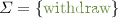and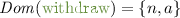, where n has a string value and a has an integer value. The string n represents a user name and the integer a represents the amount of the withdrawal by the user n. Observe that clockis never reset, and therefore measures absolute time. The automaton can non-deterministically remain in $$\ell _0$$, or start to measure a log by taking the $$\varepsilon$$-transition to $$\ell _1$$ checking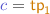, and therefore “remembering” the start time using timing parameter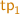. Then, whenever a user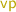has withdrawn more than half of the accumulated withdrawals (data guard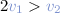) in a (50, 100) time window (timed guard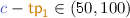), the automaton takes a $$\varepsilon$$-transition to the accepting location, checking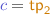, and therefore remembering the end time using timing parameter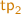.

## 6 Experiments

We implemented our symbolic monitoring algorithm in a tool SyMon in C++, where the domain for data is the strings and the integers. Our tool SyMon is distributed at https://github.com/MasWag/symon. We use PPL  for the symbolic representation of the valuations. We note that we employ an optimization to merge adjacent polyhedra in the configurations if possible. We evaluated our monitor algorithm against three original benchmarks: Copy in Fig. 1c; and Dominant and Periodic in Fig. 3. We conducted experiments on an Amazon EC2 c4.large instance (2.9 GHz Intel Xeon E5-2666 v3, 2 vCPUs, and 3.75 GiB RAM) that runs Ubuntu 18.04 LTS (64 bit).

### 6.1 Benchmark 1: Copy

Our first benchmark Copy is a monitoring of variable updates much like the scenario in . The actions are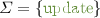and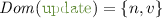, where n has a string value representing the name of the updated variables and v has an integer value representing the updated value. Our set consists of 10 timed data words of length 4,000 to 40,000.

The PTDA in Copy is shown in Fig. 1c, where we give an additional constraint $$3< \mathsf {tp}< 10$$ on $$\mathsf {tp}$$. The property encoded in Fig. 1c is “for any variable, whenever an update of that variable occurs, then within $$\mathsf {tp}$$ time units, the value of b must be equal to that update”.

The experiment result is in Fig. 4. We observe that the execution time is linear to the number of the events and the memory usage is more or less constant with respect to the number of events.

### 6.2 Benchmark 2: Dominant

Our second benchmark is Dominant (Fig. 3 left). Our set consists of 10 timed data words of length 2,000 to 20,000. The experiment result is in Fig. 5. We observe that the execution time is linear to the number of the events and the memory usage is more or less constant with respect to the number of events.

### 6.3 Benchmark 3: Periodic

Our third benchmark Periodic is inspired by a parameter identification of periodic withdrawals from one bank account. The actions are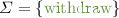and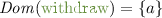, where a has an integer value representing the amount of the withdrawal. We randomly generated a set consisting of 10 timed data words of length 2,000 to 20,000. Each timed data word consists of the following three kinds of periodic withdrawals:

• $$\mathbf{short period}$$ One withdrawal occurs every $$5\pm 1$$ time units. The amount of the withdrawal is $$50\pm 3$$.

• $$\mathbf{middle period}$$ One withdrawal occurs every $$50\pm 3$$ time units. The amount of the withdrawal is $$1000\pm 40$$.

• $$\mathbf{long period}$$ One withdrawal occurs every $$100\pm 5$$ time units. The amount of the withdrawal is $$5000\pm 20$$.

The PTDA in Periodic is shown in the right of Fig. 3. The PTDA matches situations where, for any two successive withdrawals of amount more than $$\mathsf {vp}$$, the duration between them is within $$[\mathsf {tp}_1, \mathsf {tp}_2]$$. By the symbolic monitoring, one can identify the period of the periodic withdrawals of amount greater than $$\mathsf {vp}$$ is in $$[\mathsf {tp}_1, \mathsf {tp}_2]$$. An example of the validity domain is shown in the right figure.

The experiment result is in Fig. 5. We observe that the execution time is linear to the number of the events and the memory usage is more or less constant with respect to the number of events.

### 6.4 Discussion

First, a positive result is that our algorithm effectively performs symbolic monitoring on more than 10,000 actions in one or two minutes even though the PTDAs feature both timing and data parameters. The execution time in Copy is 50–100 times smaller than that in Dominant and Periodic. This is because the constraint $$3< \mathsf {tp}< 10$$ in Copy is strict and the size of the configurations ($$Conf ^{o}_{i}$$ and $$Conf ^{u}_{i}$$ in Algorithm 1) is small. Another positive result is that in all of the benchmarks, the execution time is linear and the memory usage is more or less constant in the size of the input word. This is because the size of configurations ($$Conf ^{o}_{i}$$ and $$Conf ^{u}_{i}$$ in Algorithm 1) is bounded due to the following reason. In Dominant, the loop in $$\ell _1$$ of the PTDA is deterministic, and because of the guard $$c- \mathsf {tp}_1 \in (50,100)$$ in the edge from $$\ell _1$$ to $$\ell _2$$, the number of the loop edges at $$\ell _1$$ in an accepting run is bounded (if the duration between two continuing actions are bounded as in the current setting). Therefore, $$| Conf ^{o}_{i}|$$ and $$| Conf ^{u}_{i}|$$ in Algorithm 1 are bounded. The reason is similar in Copy, too. In Periodic, since the PTDA is deterministic and the valuations of the amount of the withdrawals are in finite number, $$| Conf ^{o}_{i}|$$ and $$| Conf ^{u}_{i}|$$ in Algorithm 1 are bounded.

It is clear that we can design ad-hoc automata for which the execution time of symbolic monitoring can grow much faster (e.g., exponential in the size of input word). However, experiments showed that our algorithm monitors various interesting properties in a reasonable time.

Copy and Dominant use data and timing parameters as well as memory and aggregation; from Table 1, no other monitoring tool can compute the valuations satisfying the specification. We however used the parametric timed model checker IMITATOR  to try to perform such a synthesis, by encoding the input log as a separate automaton; but IMITATOR ran out of memory (on a 3.75 GiB RAM computer) for Dominant with, while SyMon terminates in 14 s with only 6.9 MiB for the same benchmark. Concerning Periodic, the only existing work that can possibly accommodate this specification is . While the precise performance comparison is interesting future work (their implementation is not publicly available), we do not expect our implementation be vastly outperformed: in , their tool times out (after 10 min) for a simple specification (“$$\mathbf {E}_{[0, s_2]} \mathbf {G}_{[0, s_1]} (x < p)$$”) and a signal discretized by only 128 points.

For those problem instances which MonPoly and DejaVu can accommodate (which are simpler and less parametrized than our benchmarks), they tend to run much faster than ours. For example, in , it is reported that they can process a trace of length 1,100,004 in 30.3 s. The trade-off here is expressivity: for example, DejaVu does not seem to accommodate Dominant, because DejaVu does not allow for aggregation. We also note that, while SyMon can be slower than MonPoly and DejaVu, it is fast enough for many scenarios of real-world online monitoring.

## 7 Conclusion and Perspectives

We proposed a symbolic framework for monitoring using parameters both in data and time. Logs can use timestamps and infinite domain data, while our monitor automata can use timing and variable parameters (in addition to clocks and local variables). In addition, our online algorithm can answer symbolically, by outputting all valuations (and possibly log segments) for which the specification is satisfied or violated. We implemented our approach into a prototype SyMon and experiments showed that our tool can effectively monitor logs of dozens of thousands of events in a short time.

Perspectives. Combining the BDDs used in  with some of our data types (typically strings) could improve our approach by making it even more symbolic. Also, taking advantage of the polarity of some parameters (typically the timing parameters, in the line of ) could improve further the efficiency.

We considered infinite domains, but the case of finite domains raises interesting questions concerning result representation: if the answer to a property is “neither $$\mathtt {a}$$ nor $$\mathtt {b}$$”, knowing the domain is $$\{ \mathtt {a}, \mathtt {b}, \mathtt {c} \}$$, then the answer should be $$\mathtt {c}$$.